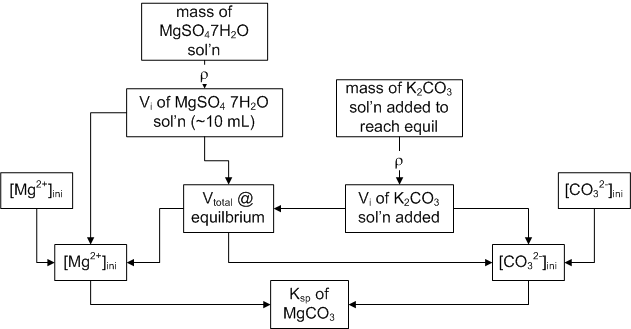Ksp of Magnesium Carbonate

You are going to try ot determine the Ksp of magnesium carbonate, which is the equilbrium constant for the reaction:

Mg2+(aq) + CO32-(aq)  MgCO3(s)

To determine that value we only need the concentartion of magnesium and carbonate ions at equilibrium. We are going to slowly add two solutions together until we get the first hint of precipitation.

Our procedure will consist of adding a K2CO3 solution drop by drop to a a solution of magsium sulfate heptahydrate until a precipitate just ‘remains’ (thereby making a saturated solution) Knowing the V (and hence concentration) of each solution at that magic point, we can determine the concentrations at equilibirum (with the solid) which allows us to calc Ksp.

Solutions you will make up:
50.00 mL (volumetric flask) of 0.015 M K2CO3 You will need to know the density of this solution, so write down the mass of empty flask before you add solid and solution.
100.00 mL (volumetric flask) of 0.004 M MgSO4·7H2O You need the density of this solution too.

Procedure: Add ~10 mL of MgSO4·7H2O to a stoppered test tube You need to know the EXACT mass of solution added. Then add K2CO3 solution drop by drop (quick and dirty run might make your day go faster) until the endpoint. To see if you are at the endpoint, shake vigorously, let the solution settle, then do a gentle inversion and then look for small particles. If you see some, you be done.

Calculation picture:Lab Report: consists of short form memo Procedure, results, commentary and attachments. Make sure you report, Ave Ksp, S.D. of Ksp, as well as % error (between your ave and the real answer
Prelab: (6 points)
1. Similar procedure as above, but calculating the Ksp of lead (II) nitrate. 10.00 mL of 0.020 M solution of lead nitrate is made. It takes exactly 0.350 mL of a 0.020 M potasium iodide solution to give ‘permenant’ precipitation. Calculate Ksp of lead iodide.
2. Calculate the mass of solid needed to make your solutions for the experiment

MEO Last Updated 31Dec10